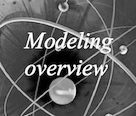# Team:EPF-Lausanne/Analysis methods

## ContentsAnalysis Methods

# Softwares used

### VMD

VMD is a molecular visualization program for displaying, animating, and analyzing biomolecular systems using 3-D graphics and built-in scripting. It provides a wide range of molecular representations, and includes tools for working with volumetric data, sequence data, and arbitrary graphics objects. You can have more information on their webpage.

### NAMD

NAMD is a molecular dynamics code designed for simulation of large biomolecular systems. It is based on Charm++ parallel programming model, and uses VMD for simulation setup and trajectory analysis. See their website here.

# Information needed

### Generating input files

In this section, we explain all the steps to create needed files for NAMD, except the .conf file, which is just below. We need a compatible .pdb in addition to parameter and topology files to go through. Steps to generate all the input files are explained in detail on this page: How to generate input files. This is a kind of summary of the tutorial.

### Launch a simulation

We start from .pdb, .psf, .rtf generated in the previous sections and we explain how to launch NAMD on both clusters we have access to. Complete process is on a separate page How to launch a simulation.

### Namd .conf parameters

Namd can run different kind of simulations, from minimization to MD simulations. Here are the .conf file we used. NamdConf

### Scripts used

We stored all our scripts, which were highly modified compared to the original ones, in order to fit our needs. You can find them on this page.

# Step by step analysis

The following section is a kind of tutorial, which describes step by step how to obtain our different results.

This analysis will check whether the minimization, the heating and the equilibration took place correctly and whether the protein did not explode.

## Maxwell-Boltzmann Energy Distribution

Here we will conﬁrm that the kinetic energy distribution of the atoms in a system corresponds to the Maxwell distribution for a given temperature.

## Energies

Here we will calculate the average of various energies such as kinetic energy and different internal ones so called bonded energies (bonds, angles and dihedrals). Moreover, we will calculate non-bonded energy (electrostatic, van der Waals)) over the course of the equilibration.

## Temperature distribution

Here we will analyze the temperature distribution over the simulation. The temperature might increase linearly during the heating step and then it might remain stable.

We obtained the expected results.

## Density

Here we will analyze the behavior of the protein density over the simulation. Normally during the equilibration step density might remain more or less stable. Particularly during the NVT equilibration the density might remain constant.

We obtained the expected results.

## Pressure as a function of simulation time

Here we will analyze the pressure behavior along the minimization and the equilibration. This quantity might stabilize after the heating and remain more or less stable during the equilibration. Particularly during the NPT steps the pressure might be strictly constant.

We obtained the expected results.

## RMSD for individual residues

Here we will calculate the RMSD for each residue to determine which residue move the most. This analysis will help us to see which residue is more or less stable. After that we will try after that to select the amino acids to mutate in order to stabilize the light activated state of our LOV domain.

## RMSD of selected atoms compared to initial position along time

Here we will analyze the RMSD of each atom to check whether the protein remains more or less stable during the equilibration.

## Salt bridges

Salt bridges are non-bonded interactions between charged residues.

Here we will analyze the evolution of these interactions over the equilibration in order to check how these interactions change over the equilibration.

## RMSF

This is quite similar to the RMSD analysis. Here we will analyze how the RMSF vary for each residues.

## Angles

This part is made to measure the angle between two chains. The procedure is described below.

## H bonds and distance measurments

This part aim at finding characteristic distances, in particular for H-bonds.

## Dihedral angles

Dihedral angles measure angle between four atoms.